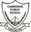Trending ▼   ResFinder# ICSE Class X Prelims 2020 : Computer Applications (Cambridge Public School, Bangalore) : Kisa paper computer

4 pages, 36 questions, 0 questions with responses, 0 total responses,20Ayyub Khan Cambridge Public School, Bangalore
+Fave Message
 Home > arshad_khan_6704 >   F Also featured on: School Page icseFormatting page ...

KARNATAKA ICSE SCHOOLS ASSOCIATION PREPARATORY EXAMINATION - JANUARY 2020 CLASS : X SUBJECT: COMPUTER APPLICATIONS MAX. MARKS : 100 DURATION : 2 HOURS DATE : ___.___. 2020 This paper is divided into two sections. Attempt all questions from Section A and any four questions from Section B. The intended marks for questions or parts of questions are given in brackets [] SECTION A (40 MARKS) (Attempt all questions) [5x2=10] Question1 a) What is polymorphism? b) Write the number of bits allocated for char x[][] of 3rows and 2 columns. c) What are static members? d) Write the return data type of i) compareToIgnoreCase() ii) parseLong() e) Differentiate between break and System. exit(0) Question2 a) Rewrite the following using ternary operator: int n=27;String s=""; if(n>=18) s="eligible"; else s="not eligible"; b) What is meant by private visibility of a method? c) Give the difference between pure and impure function. d) What is an exception? Write one example. e) Explain autoboxing with an example. [5x2=10] Question3 a) Evaluate the following Java expression if a=4 andb=6 a+=++b%a--+a/2+a [10x2=20] b) Write valid Java statement for the following: d =|x| - c) Write the output for the following: i)System.out.println(Math.round(8.3)); ii)System.out.println(Math.ceil(Math.min(Math.PI,17.7))); d) int a[][] = {{1, 0, 0},{0, 1, 0}, {0, 0, 1},{0,1,0}}; System.out.println(a.length); System.out.println(a.length); 1Formatting page ...Formatting page ...Formatting page ...